Question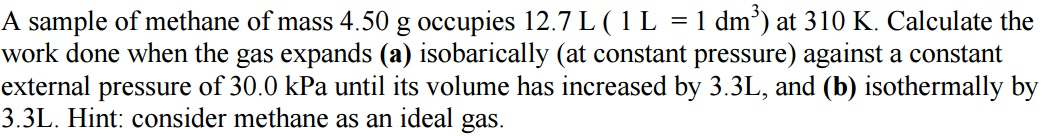given data,

m = 4.5 g

V1 = 12.7 L = 12.7*10^-3 m^3

T = 310 k

a) In Isobaric process pressure remains cosnatnt.

here, V2 = 12.7 + 3.3 L

= 16 L

= 16*10^-3 m^3

P = 30 kPa = 3*10^4 pa

Workdone in Isobaric process,
W = P*(V2 - V1)

= 3*10^4*3.3*10^-3

= 99 J

b) In Isothemrla process Temperature remains constant.

no of moles, n = mass/molar mass

= 4.5/16

= 0.28125 mole

Workdone in Isothermal process,
W = n*R*T*ln(V2/V1)

= 0.28125*8.314*310*ln(16/12.7)

= 167.4 J

#### Earn Coins

Coins can be redeemed for fabulous gifts.

Similar Homework Help Questions
• ### A sample of 4.50 g of methane occupies 12.7 L at 310 K. a) calculate the...

A sample of 4.50 g of methane occupies 12.7 L at 310 K. a) calculate the work done when the gas expands isothermally against a constant external pressure of 200 torr until its volume has increased by 3.3 L. B) calculate the work that would be done if the same expansion occurred reversibly.

• ### 3. A sample of argon of mass 3.56 g occupies 12.5 dm at 305 K. (a)...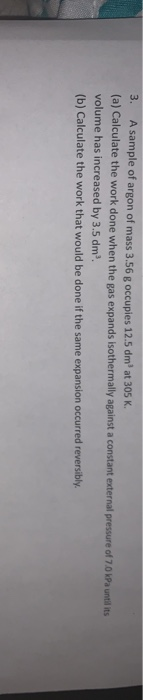3. A sample of argon of mass 3.56 g occupies 12.5 dm at 305 K. (a) Calculate the work done when the gas expands isothermally against a constant external pressure of 70 kPa utilit volume has increased by 3.5 dm. (b) Calculate the work that would be done if the same expansion occurred reversibly.

• ### An ideal gas with γ=1.4 occupies 5.0 L at 300 K and 100 kPa pressure and...

An ideal gas with γ=1.4 occupies 5.0 L at 300 K and 100 kPa pressure and is heated at constant volume until its pressure has doubled. It's then compressed adiabatically until its volume is one-fourth its original value, then cooled at constant volume to 300 K , and finally allowed to expand isothermally to its original state. Find the net work done on the gas in Joules.

• ### 2) Consider a sample of gas that occupies 23.1 L at 1.13 atm. What volume will...

2) Consider a sample of gas that occupies 23.1 L at 1.13 atm. What volume will the same sample occupy if the pressure is increased to 3.05 atm while the temperature is held constant? 3) A fixed sample of gas occupies 25.9 mL at 211.3 K. What volume will the gas occupy at 336.6 K? 4) A fixed sample of gas exerts a pressure of 580 Torr at 284 K. What will the pressure be (in atm) if the temperature...

• ### A sample of methane gas that occupies a volume of 45.5 L at a temperature of...

A sample of methane gas that occupies a volume of 45.5 L at a temperature of 0 °C and a pressure of 1 atm contains __ moles of gas.

• ### A sample of methane gas at a pressure of 1.17 atm and a temperature of 23.8...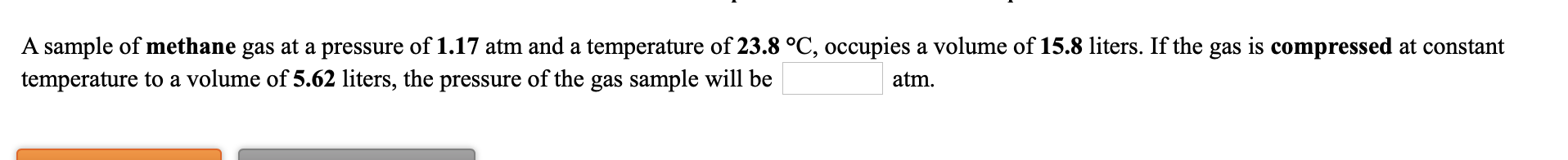A sample of methane gas at a pressure of 1.17 atm and a temperature of 23.8 °C, occupies a volume of 15.8 liters. If the gas is compressed at constant temperature to a volume of 5.62 liters, the pressure of the gas sample will be atm. A sample of oxygen gas at a pressure of 0.976 atm and a temperature of 22.7 °C, occupies a volume of 786 mL. If the gas is allowed to expand at constant temperature until...

• ### Part A ConstantsI Periodic Table An ideal gas with γ = 1.4 occupies 6.0 L at...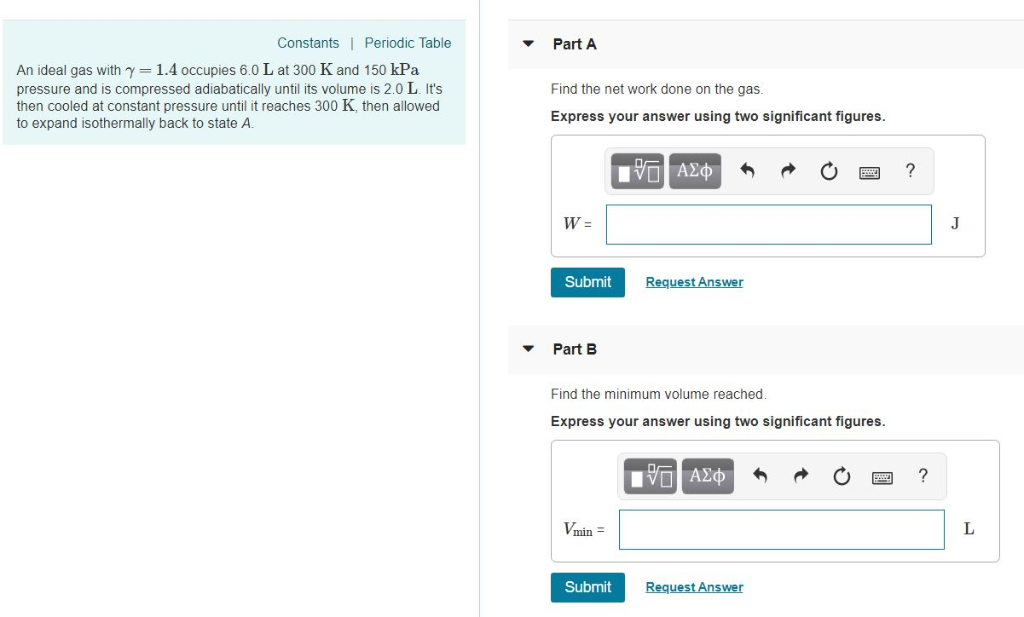Part A ConstantsI Periodic Table An ideal gas with γ = 1.4 occupies 6.0 L at 300 K and 150 kPa pressure and is compressed adiabatically until its volume is 2.0 L. It's then cooled at constant pressure until it reaches 300 K, then allowed to expand isothermally back to state A. Find the net work done on the gas Express your answer using two significant figures. 0図? W- Submit Request Answer Part B Find the minimum volume reached Express...

• ### A sample of a monatomic ideal gas occupies 5.00 L at atmospheric pressure and 300 K...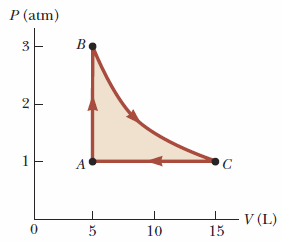A sample of a monatomic ideal gas occupies 5.00 L at atmospheric pressure and 300 K (point A in the figure below). It is warmed at constant volume to 3.00 atm (point B). Then it is allowed to expand isothermally to 1.00 atm (point C) and at last compressed isobarically to its original state. a. Find Q, W, and ΔEint for each of the processes. Q (kJ) W (kJ) Eint (kJ) A → B B → C C → A...

• ### A sample of a monatomic ideal gas occupies 5.00 L at atmospheric pressure and 300 K...A sample of a monatomic ideal gas occupies 5.00 L at atmospheric pressure and 300 K (point A in the figure below). It is warmed at constant volume to 3.00 atm (point B). Then it is allowed to expand isothermally to 1.00 atm (point C) and at last compressed isobarically to its original state. (a) Find the number of moles in the sample. moles (b) Find the temperature at point B. K (c) Find the temperature at point C. K...

• ### An ideal gas with ?=1.4 occupies 5.5L at 300 K and 150kPa pressure and is compressed...

An ideal gas with ?=1.4 occupies 5.5L at 300 K and 150kPa pressure and is compressed adiabatically until its volume is 2.0 L. It's then cooled at constant pressure until it reaches 300 K, then allowed to expand isothermally back to state A. A)Find the net work done on the gas B) Find the minimum volume reached.